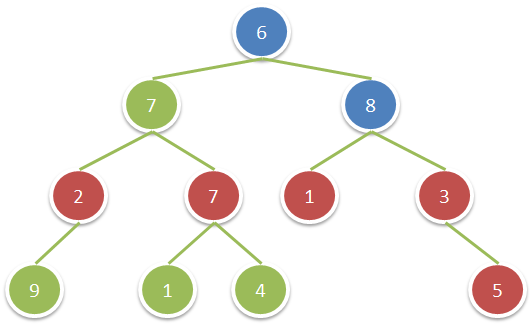GeetCode Hub

Given a binary tree, return the sum of values of nodes with even-valued grandparent.  (A grandparent of a node is the parent of its parent, if it exists.)

If there are no nodes with an even-valued grandparent, return 0.

Example 1:Input: root = [6,7,8,2,7,1,3,9,null,1,4,null,null,null,5]
Output: 18
Explanation: The red nodes are the nodes with even-value grandparent while the blue nodes are the even-value grandparents.

Constraints:

• The number of nodes in the tree is between 1 and 10^4.
• The value of nodes is between 1 and 100.

/** * Definition for a binary tree node. * public class TreeNode { * int val; * TreeNode left; * TreeNode right; * TreeNode() {} * TreeNode(int val) { this.val = val; } * TreeNode(int val, TreeNode left, TreeNode right) { * this.val = val; * this.left = left; * this.right = right; * } * } */ class Solution { public int sumEvenGrandparent(TreeNode root) { } }